CipherDec 16, 2016

Adam Weishaupt - Founder of Bavarian Illuminati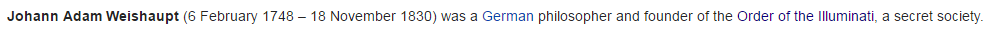Raised by Jesuits of course, how nice to see them involved right?
The order was first known by these 2 names..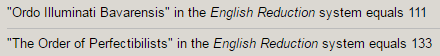'Ordo Illuminati Bavarensis' = 119 with the S exception,  like their Symbol - the All seeing eye (Also known as the eye of providence)
All Seeing Eye = 119, Star of David = 119, Master plan = 119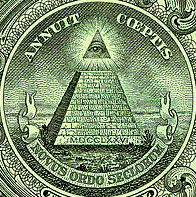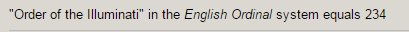234+432 = 666, supposed tilt of the earth is 23.4° degrees leaving 66.6° degrees out of 90°

Adam Weishaupt -  Born - 2/6/1748 - 2+6+1+7+4+8 = 28 (Within the Order he was called 'Brother Spartacus' - 69, Spartacus = 28, Brother = 41 , Weishaupt = 41 = 13th prime, 13 families, 13 colonies)
2/6 - Johann = 26

Died Eighteenth of November 1830 in Gotha (Gotha = 51, Adam Weishaupt = 51)6x6x6 = 216

11/18/1830 - 1+1+1+8+1+8+3 = 23
11/18/1830 - 11+18+18+30 = 77 ,  Christ = 77, Judaism = 77 (he was born in Jewish family)
11/18/30 - 11+18+30 = 59May 1st 1776 and the day of his father's sudden death have perfectly matching gematria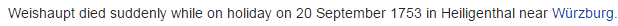9/20/1753 - 9+2+1+7+5+3 = 27
9/20/1753 - 9+20+17+53 = 99
9/20/53 - 9+20+53 = 82

5/1/1776 - 5+1+1+7+7+6 = 27 (Order was founded in 'Bavaria' = 27)
5/1/1776 = 5+1+17+76 = 99 (Thirteen = 99) 13 families founded the Order originally
5/1/76 = 5+1+76 = 82 (Adam Weishaupt died at the age of 82)
His father supposedly  "Died suddenly" while on 'Holiday' = 74 - 'September Twentieth' = 227 (22:7 = 3.14) Twenty Two divided by Seven = 314, 'Weapons of Mass destruction' = 314

Johann Georg Weishaupt died in Wurzburg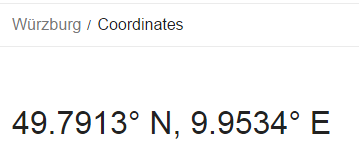49°N - 49th prime number is 227 (September Twentieth = 227)His father was a 'Lawyer' = 84',  born in 'Arnsberg' = 84/39  (Westphalia = 42 E. Reduction)51° North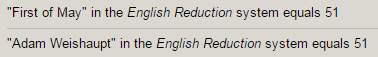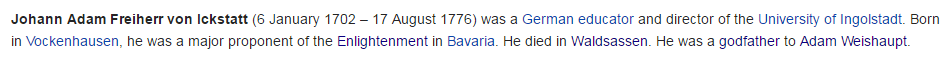Adam Weishaupt was raised by his godfather who died in August 1776, 44 days after Declaration of Independence on July 4th 17768/17/1776 - 8+17+17+76 = 118Also 1 month and 13 days which looks a lot like 113

Measuring from creation of Illuminati on 5/1/1776 to 7/4/1776 is 64 days (64th prime is 311 a reflection of 113)Israel = 64, Zion = 64, Thelema = 64, Do What thou wilt = 64, Synagogue of Satan = 64
From 5/1/1776 to 8/17/1776 is a span of 108 days, 'Order of the Illuminati' - 108